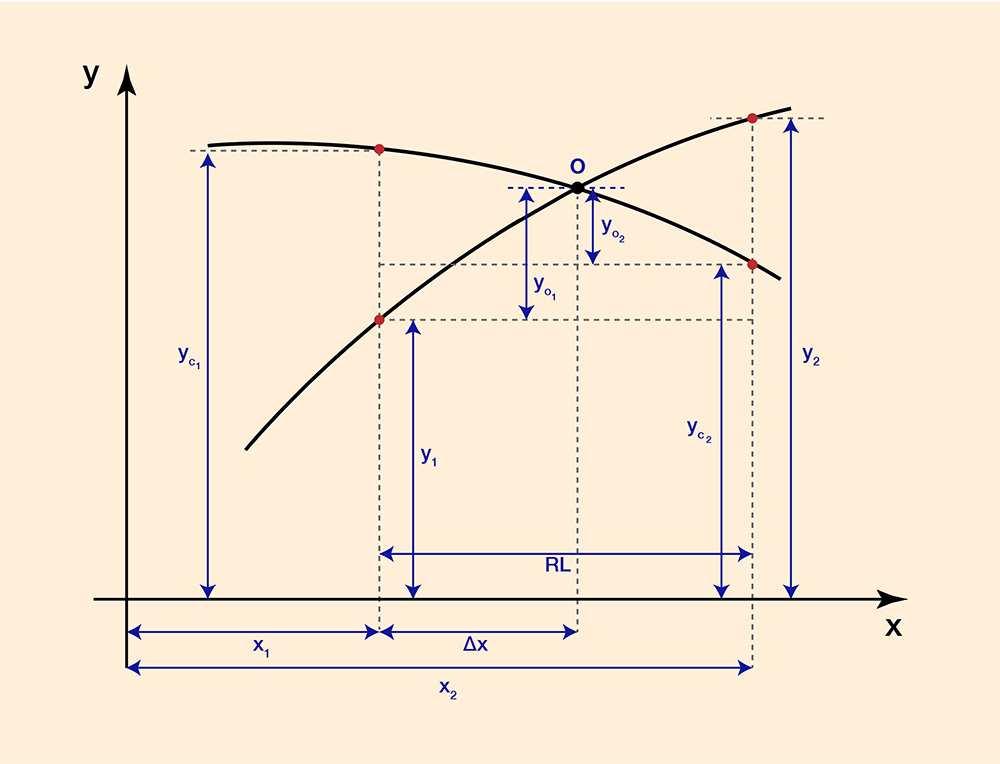Formulas to calculate the coordinates xo and yo of the intersection O of two curves y = f1 (x) and yc = f2 (x), given the ordinates of two (2) points per curve (red points), located near the intersection O, with one abscissa at x1, to the left of point O, and the other abscissa at x2, to the right of point O: y1 = f1 (x1); y2 = f1 (x2); yc1 = f2 (x1); and yc2 = f2 (x2); reach length RL = x2 - x1

[The accuracy of the calculation increases with decreasing value of the interval x2 - x1].Fig. 1  The intersection of two curves on the x-y plane.

 yo1           y2 - y1             y2 - y1 ____    =    ________     =     ________  Δx           x2 - x1                RL (1)

 y2 - y1 yo1  =   __________  Δx                 RL (1a)

 yo2                yc1 - yc2 __________    =   ___________   RL - Δx               RL (2)

 yc1 - yc2 yo2  =   ___________  (RL - Δx)                  RL (2a)

 yo1 -  yo2   =   yc2 -  y1 (3)

 y2 - y1                yc1 - yc2 _________  Δx   -   ___________  (RL - Δx)   =   yc2 -  y1     RL                       RL (3a)

 RL (yc2 - y1)   =   (y2 - y1) Δx  -  (yc1 - yc2) (RL - Δx) (3b)

 (y2 -  y1) Δx  +  (yc1 - yc2) Δx   =   RL (yc2  -   y1)  +  RL (yc1 - yc2) (3c)

 Δx (y2 - y1 + yc1 - yc2)   =   RL (yc1 - y1) (3d)

 yc1 - y1 Δx   =   RL  _____________________                      y2 - y1 + yc1 - yc2 (3e)

 Δx yo   =   y1 + yo1   =   y1 + _____ (y2 - y1)                                          RL (4)

 Δx yo   =   y1 + _____ (y2 - y1)                      RL (5)

 xo   =   x1 +  Δx (6)

These formulas are used to calculate the location where critical depth equals normal depth in the online calculator ONLINE TRANSITION DESIGN SUPERCRITICAL B.

 180905 15:45